Optimization

Optimization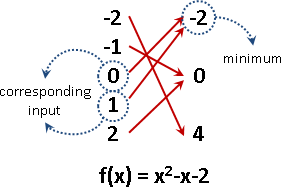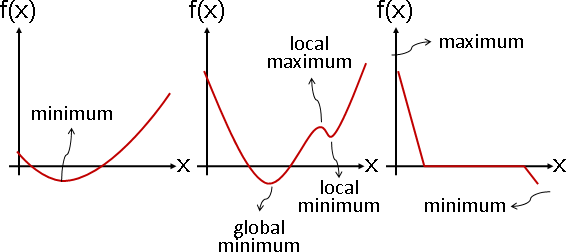Unimodal Function：單峰函數。先遞增、再遞減。只有遞增或者只有遞減，也算是單峰函數。適合Trisection Method。

Polynomial Function：多項式函數。「斜率等於零」的地方是極值、鞍點。可以手工推導公式解，也可以使用最佳化演算法求解。

Optimization - 前後包夾類型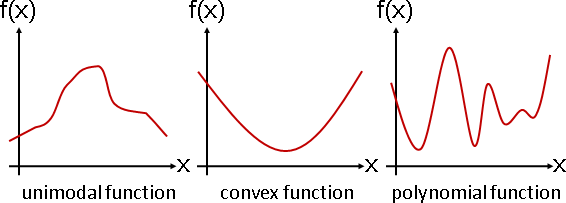Bisection Method（二分法）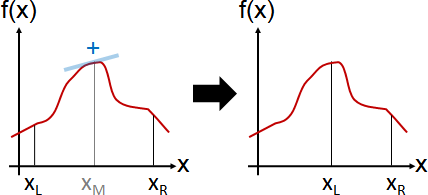dx和df已經不再符合數學家的定義。重新標記為Δx和Δf。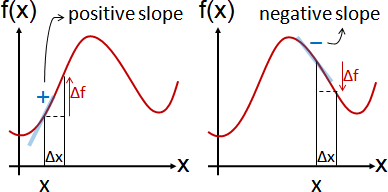Trisection Method（三分法）UVa 1476 10385 11243 11562 11613

Golden Section Method（黃金分割法）ψ是黃金比率的倒數。ψ = 1/φ = (√̅5 - 1) / 2 = 0.618...。

Ensemble Averaging（總體平均）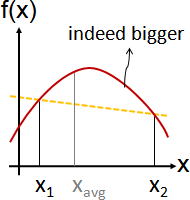Optimization - 孤軍深入類型```牛頓法求根　　　　　xnext = x - f(x)  / f′(x)

```

Newton's Method（牛頓法）

Quasi-Newton Method（擬牛頓法）（類牛頓法）

Fixed Point Iteration（不動點遞推法）

Optimization - 地面勘查類型Show[ Plot3D[BesselJ[0, Norm[{x, y}]], {x, -4Pi, 4Pi}, {y, -4Pi, 4Pi}, PlotRange -> {-1, 1}, Boxed -> False, Mesh -> None, Axes -> False, PlotPoints -> 50, ColorFunction -> (ColorData["CherryTones"][Rescale[#3, {-4, 4}]] &)], ParametricPlot3D[{{-5, u, BesselJ[0, Norm[{-5, u}]]}, {u, -8, BesselJ[0, Norm[{u, -8}]]}}, {u, -4Pi, 4Pi}, PlotStyle -> {Red, Red}] ]
ParametricPlot3D[{v Cos[u], v Sin[u], 5 Cos[Pi/10] BesselJ[0, v]}, {u, 0, 4 (Pi/2)}, {v, 0, 15}, Boxed -> False, Axes -> False, Mesh -> None]

Hill Climbing（登山法）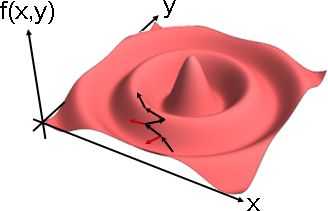Simulated Annealing（模擬退火法）UVa 10228

X座標、Y座標、……分開處理，互不干涉。當前位置，求得相鄰高度差，座標大的高度減去座標小的高度，正負值可判別相對高低。當前位置座標，加上相鄰高度差，就是往上走。若相鄰高度差越多，則前後座標差越多。坡越陡、跨越遠、走越快。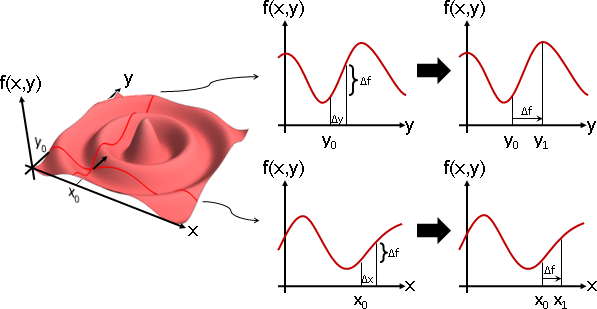Plot[BesselJ[0, Norm[{-5, y}]], {y, -4Pi, 4Pi}, PlotRange -> {-1,1}] Plot[BesselJ[0, Norm[{x, -8}]], {x, -4Pi, 4Pi}, PlotRange -> {-1,1}]Line Search（直線搜尋）Steepest Descent（最陡下降法）

Coordinate Descent（座標下降法）

Optimization - 空中勘查類型

Particle Swarm Optimization（粒子演算法）

```一、散佈N個粒子。一個粒子對應一個可行解。
position[1...N];	// x
solution[1...N];	// f(x)

然後移動粒子，讓粒子飛往最佳解。
velocity[i] =
2 * rand(0 ~ 1) * (best_position[i] - position[i]) +
2 * rand(0 ~ 1) * (best_position[best_particle_index] - position[i]);

best_solution[i] = max(best_solution[i], solution[i])
and record best_position[i];
best_particle_solution = max_arg(best_solution[1...N])
and record best_particle_index;

```

Bee Colony Optimization（蜜蜂演算法）

```一、散佈N個食物。一個食物對應一個可行解。
position[1...N];	// x
solution[1...N];	// f(x)

position[i] +=
rand(-1 ~ +1) * position[rand(1 ~ N except i)];

probability[i] = solution[i] / ( solution + ... + solution[N] )
返回時腦中記得的位置一樣會有偏差。公式同二。

隨機散佈食物，補足食物直到N個。

```

Optimization - 排列組合類型

Genetic Algorithm（基因演算法）

```1.
[初始化]

1010101010
1011001011
1110101011
0010101000

2.
[fitness function]

f(1010101010) = 678

3.
[selection]

1010101  010
1011001  011
1110101  011
0010101  000

4.
[crossover]

1010101 \/ 010  ->  1010101 -- 011
1011001 /\ 011      1011001 -- 010

1010101011
1011001010
1110101010
1010101000

5.
[mutation]

1010111011
1011001000
1110101010
1010101001

6.

```
```1. 隨機產生N個x。
2. 計算fitness function。
3. 重複以下步驟，直到有一個x讓人滿意。
甲、selection。
乙、crossover。
丙、mutation。
丁、計算fitness function。
```

```N個物品。選出其中幾個。

[初始化]

0代表對應的物品不在背包內，
1代表對應的物品在背包內。

[fitness function]

```

UVa 10715

```E條邊。選出V-1條。

[初始化]

0代表對應的邊不是MST。
1代表對應的邊是MST。

[fitness function]

```

```N個城市，C(N,2)條路。選出N條。

[初始化]

[fitness function]

[crossover]

[mutation]

1. 隨便交換兩個數字。
2. 隨便找一段數字，顛倒前後順序。
3. 隨便拿出一個數字，隨便插入到其他地方。
```

Ant Colony Optimization（螞蟻演算法）

```1.
[初始化]

2.
[state transition rule]

◎探索：隨便走一條路。機率為q。
◎追蹤：走費洛蒙最強的道路。機率為1-q。

q是螞蟻選擇探索的機率，自行設定在0到1之間。

3.
[local updating rule]

◎自然蒸發，餘下：原本費洛蒙值，乘上1-ρ。
◎螞蟻路過，添加：道路起點所連接的道路當中，最大的那個費洛蒙值，乘上p。

ρ是費洛蒙蒸發的程度，自行設定在0到1之間。

4.

5.
[global updating rule]
N隻螞蟻回巢之後，

◎自然蒸發，餘下：原本費洛蒙值，乘上1-α。

◎最佳路線，添加：目前最佳解的數值，倒數後，再乘上α。

α是費洛蒙蒸發的程度，自行設定在0到1之間。

6.
2. 3. 4. 5.，重複執行R次。

```
```1. 初始化地圖與費洛蒙。
2. 以下動作執行R次：
1. N隻螞蟻依序找路線，不是同時找路線：
1. state transition rule，一隻螞蟻找一條路線。
此路線是由蟻窩出發，經過所有食物各一次，然後回到蟻窩。
2. 記錄目前最佳路線。
3. local updating rule，更新路線費洛蒙。
2. global updating rule，更新所有道路費洛蒙。
```

```N個城市，C(N,2)條路。選出N條。

[初始化]

```

Optimization - 複雜網路類型

Constrained Optimization

Constrained Optimization

「約束最佳化」。最佳化，限制輸入範圍。

```maximize f(x)
x ∈ C
```

「約束最佳化」。最佳化、等式與不等式組，兩者合體。

```maximize f(x)   subject to g(x) = 0, h(x) ≥ 0, ...
```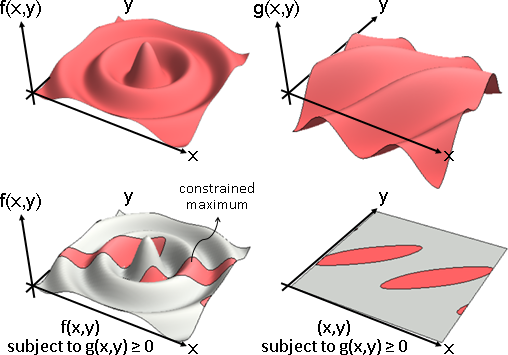Show[ Plot3D[(Cos[(x-y)/2 - 3] + Cos[y/4])/2 - 1/2, {x, -4Pi, 4Pi}, {y, -4Pi, 4Pi}, PlotRange -> {-1, 1}, Boxed -> False, Mesh -> None, Axes -> False, PlotPoints -> 50, ColorFunction -> (ColorData["CherryTones"][Rescale[#3, {-4, 4}]] &)], ParametricPlot3D[{{u,-4Pi,0}, {-4Pi,u,0}}, {u, -4Pi, 4Pi}, PlotStyle -> {Black, Black}] ]
Show[ Plot3D[BesselJ[0, Norm[{x, y}]], {x, -4Pi, 4Pi}, {y, -4Pi, 4Pi}, PlotRange -> {-1, 1}, Boxed -> False, Mesh -> None, Axes -> False, PlotPoints -> 50, ColorFunction -> (ColorData["GrayTones"][Rescale[#3, {-6, 2}]] &)], Plot3D[BesselJ[0, Norm[{x, y}]] + 0.01, {x, -4Pi, 4Pi}, {y, -4Pi, 4Pi}, PlotRange -> {-1, 1}, Boxed -> False, Mesh -> None, Axes -> False, PlotPoints -> 50, RegionFunction -> Function[{x, y, z}, Cos[(x-y)/2 - 3] + Cos[y/4] > 1], ColorFunction -> (ColorData["CherryTones"][Rescale[#3, {-4, 4}]] &)] ]
Show[ Plot3D[0, {x, -4Pi, 4Pi}, {y, -4Pi, 4Pi}, PlotRange -> {-1, 1}, Boxed -> False, Mesh -> None, Axes -> False, PlotPoints -> 50, ColorFunction -> (ColorData["GrayTones"][Rescale[#3, {-6, 2}]] &)], Plot3D[0.01, {x, -4Pi, 4Pi}, {y, -4Pi, 4Pi}, PlotRange -> {-1, 1}, Boxed -> False, Mesh -> None, Axes -> False, PlotPoints -> 50, RegionFunction -> Function[{x, y, z}, Cos[(x-y)/2 - 3] + Cos[y/4] > 1], ColorFunction -> (ColorData["CherryTones"][Rescale[#3, {-4, 4}]] &)] ]
ContourPlot[(Cos[(x-y)/2 - 3] + Cos[y/4])/2 - 1/2 == 0, {x, -4Pi, 4Pi}, {y, -4Pi, 4Pi}]

Feasible Direction Method（可行方向法）

```p = argmin [ ∇f(x) dot (p - x) ] = argmin [ ∇f(x) dot p ]
p∈C                            p∈C

xnext = x + step ⋅ (p - x)
```

```p = x + step ⋅ ∇f(x)

xnext = argmin ‖ p - xnext ‖
xnext∈C
```

Lagrange Multiplier（拉格朗日乘數）

```f(x,y) 求極值。必須滿足 g(x,y) = 0。

f(x,y) 的極值，等同 L(x,y,λ) = f(x,y) + λ g(x,y) 的極值。

{ ∂/∂x L(x,y,λ) = 0
{ ∂/∂y L(x,y,λ) = 0
{ ∂/∂λ L(x,y,λ) = 0

∇L(x,y,λ) = 0
```

L(x,y,λ)稱作「拉格朗日函數Lagrangian」。λ稱作「拉格朗日乘數Lagrange Multiplier」。

```∇L(x,y,λ) = 0        梯度等於零、求駐點。

max L(x,y,λ)         ✘ 注意到，求駐點，不等價於求極值。
x,y,λ
max min L(x,y,λ)     ✘ 注意到，求駐點，不等價於求鞍點。
x,y   λ
```

```三式分別偏微分。
{ ∂/∂x L(x,y,λ) = 0
{ ∂/∂y L(x,y,λ) = 0
{ ∂/∂λ L(x,y,λ) = 0

{ ∂/∂x f(x,y) + λ ⋅ ∂/∂x g(x,y) = 0
{ ∂/∂y f(x,y) + λ ⋅ ∂/∂y g(x,y) = 0
{ g(x,y) = 0

∇f(x,y) + λ ∇g(x,y) = 0
∇f(x,y) = -λ ∇g(x,y)

∇f(x,y) = λ ∇g(x,y)

{ ∇f(x,y) = λ ∇g(x,y)
{ g(x,y) = 0
```

```f(x,y) 求極值。必須滿足 g₁(x,y) = 0 與 g₂(x,y) = 0 與……。

```

Karush–Kuhn–Tucker Conditions（KKT條件）

```maximize f(x)   subject to g(x) = 0

---> solve { ∇f(x) = λ ∇g(x)
{ g(x) = 0
```

```maximize f(x)   subject to h(x) ≥ 0

---> solve { ∇f(x) = μ ∇h(x)
{ μ ≤ 0
{ h(x) ≥ 0
```

```                      | ∇f(x) = μ ∇h(x) | ∇f(x) + μ ∇h(x) = 0
----------------------|-----------------|---------------------
max f(x) st h(x) ≥ 0  | μ ≤ 0           | μ ≥ 0
max f(x) st h(x) ≤ 0  | μ ≥ 0           | μ ≤ 0
min f(x) st h(x) ≥ 0  | μ ≥ 0           | μ ≤ 0
min f(x) st h(x) ≤ 0  | μ ≤ 0           | μ ≥ 0
```

```maximize f(x)   subject to g₁(x) = 0, g₂(x) = 0, h₁(x) ≥ 0, h₂(x) ≥ 0

---> solve { ∇f(x) = λ₁ ∇g₁(x) + λ₂ ∇g₂(x) + μ₁ ∇h₁(x) + μ₂ ∇h₂(x)
{ g₁(x) = 0
{ g₂(x) = 0
{ μ₁ ≤ 0
{ h₁(x) ≥ 0
{ μ₂ ≤ 0
{ h₂(x) ≥ 0
```

Penalty Method（懲罰法）

```     maximize f(x)               subject to g(x) = 0
---> maximize f(x)               subject to g(x) = 0 and ρ/2 g(x)² = 0
---> maximize f(x) + ρ/2 g(x)²   subject to g(x) = 0
```

Barrier Method（屏障法）

```     minimize f(x)                 subject to h(x) ≥ 0
---> minimize f(x)                 subject to h(x) ≥ 0 and
-ρ log(h(x)) ≥ ∞ and ρ ≥ 0
---> minimize f(x) - ρ log(h(x))   subject to h(x) ≥ 0 and ρ ≥ 0
```

Alternating Direction Method of Multipliers（交替方向乘數法）

```maximize f(x)   subject to g(x) = 0, h(x) ≥ 0
L(x,λ,μ) = f(x) + λ g(x) + μ h(x)   where μ ≥ 0
```
```xnext = argmax { f(x) + λ g(x) + μ h(x) }
λnext = λ + step ⋅ g(x)
μnext = max(μ + step ⋅ h(x), 0)
```

```maximize f(x)   subject to Ax ≤ b
L(x,μ) = f(x) + μᵀ (Ax - b)   where μ ≤ 0
```
```xnext = argmax { f(x) + μᵀ (Ax - b) }
μnext = min(μ + step ⋅ (Ax - b), 0)
```

```minimize f(x) + g(y)   subject to Ax + By = c
L(x,y,λ) = f(x) + g(y) + λᵀ (Ax + By - c) + (ρ/2) ‖Ax + By - c‖²
```
```xnext = argmin L(x,y,λ)
ynext = argmin L(x,y,λ)
λnext = λ + step ⋅ (Ax + By - c)
```

Interior Point Method（內點法）

Multiobjective Optimization

Multiobjective Optimization

「多目標最佳化」。找到一個輸入，同時最佳化多個函數。

```{ minimize f(x)
{ maximize g(x)
{ maximize h(x)
```

Scalarization（純量化）

```{ minimize f(x)   Mencius bear paw
{ maximize g(x)  ------------------>  maximize g(x)
{ maximize h(x)
```

```{ minimize f(x)   f,g,h are convex
{ minimize g(x)  ------------------>  minimize f(x) + g(x) + h(x)
{ minimize h(x)
```

```minimize α f(x) + β g(x) + γ h(x)  (α ≥ 0, β ≥ 0, γ ≥ 0)
```

Regularization（正則化）

```minimize f(x) + α g(x) + β h(x) + ...   (α ≥ 0, β ≥ 0, ...)
```

f(x)是原本的目標，g(x) h(x) ...是追加的目標。

Regularization ≠ KKT Conditions

```Regularization:
minimize f(x) + α g(x) + β h(x) + ...   (α ≥ 0, β ≥ 0, ...)

KKT Conditions:
∇[f(x) + λ g(x) + μ h(x) + ...] = 0     (μ ≥ 0, h(x) ≥ 0, ...)
```
```Regularization: α, β, ... are known parameters
KKT Conditions: λ, μ, ... are unknown arguments
```

Regularization與KKT Conditions沒有任何關聯，只不過是數學式子有點像。別忘了KKT Conditions還得聯立其他式子。

Operator Splitting Method（算子分裂法）

```minimize f(x)  --->  minimize f₁(x) + f₂(x) + f₃(x)
where f₁(x) + f₂(x) + f₃(x) = f(x)
```
```x₁ = x₀ - ∇f₁(x)
x₂ = x₁ - ∇f₂(x)
x₃ = x₂ - ∇f₃(x)
x₄ = x₃ - ∇f₁(x)
x₅ = x₄ - ∇f₂(x)
:       :
```

Domain Decomposition Method（領域分解法）

```minimize f(x,y,z)  --->  minimize f₁(x,z) + f₂(y,z)
```

Disciplined Optimization

Disciplined Optimization

「紀律最佳化」。依照函數性質，採取適當的最佳化演算法。

Online Optimization

Online Optimization

「在線最佳化」。函數時時變化，有如波浪，隨波逐流。

Plot3D[(Sin[x/2-y] + Sin[y/4])/2, {x, -4Pi, 4Pi}, {y, -4Pi, 4Pi}, PlotRange -> {-1, 1}, Boxed -> False, Mesh -> None, Axes -> False, PlotPoints -> 50, ColorFunction -> (ColorData["CherryTones"][Rescale[#3, {-4, 4}]] &)]

Mean Shift（平均數移動）

```一、均勻散布粒子。

取得鄰近範圍內所有粒子。（範圍自訂，通常是規定半徑長度。）

```

Particle Filter（粒子濾波器）

```一、以上次的極值座標為中心，
散布n個粒子，呈高斯分布。（範圍自訂，範圍即變異數）
粒子不用飛來飛去了，直接散布。

想像n個粒子各有磁場（高斯分布），強弱由函數值大小決定，
形成joint distribution（高斯混和分布）。
下一回合依此分布散布粒子。
```